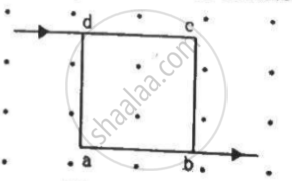Advertisement Remove all ads

# A Current of 2 a Enters at the Corner D of a Square Frame Abcd of Side 20 Cm and Leaves at the Opposite Corner B. a Magnetic Field B = 0.1 T Exists in the Space - Physics

Sum

A current of 2 A enters at the corner d of a square frame abcd of side 20 cm and leaves at the opposite corner b. A magnetic field B = 0.1 T exists in the space in a direction perpendicular to the plane of the frame, as shown in the figure. Find the magnitude and direction of the magnetic forces on the four sides of the frame.Advertisement Remove all ads

#### Solution

Given:
A square frame abcd of side, = 20 cm
Electric current through the wire, I = 2 A
Magnetic field, B = 0.1 T
The direction of magnetic field is perpendicular to the plane of the frame, coming out of the plane.
As per the question, current enters at the corner d of the square frame and leaves at the opposite corner b.
Angle between the frame and magnetic field, θ = 90˚
Magnetic force,
vecF = i vecl xx vecB
For wire da and cb,
|vecF| = ilBsin theta
|vecF| = ilBsin90°
= 2/2 xx 20 xx 10^-2xx 0.1
= 0.02 N
The direction of force can be found using Fleming's left-hand rule.
Thus, the direction of magnetic force is towards the left.
For wires dc and ab,
|vecF| = ilBsintheta
∴ |vecF| = ilBsin90^circ
= 2/2 xx 20 xx 10^-2xx0.1
= 0.02 N
The direction of force can be found using Fleming's left-hand rule.
Thus, the direction of magnetic force is downwards.

Concept: Force on a Moving Charge in Uniform Magnetic and Electric Fields
Is there an error in this question or solution?
Advertisement Remove all ads

#### APPEARS IN

HC Verma Class 11, Class 12 Concepts of Physics Vol. 2
Chapter 12 Magnetic Field
Q 8 | Page 230
Advertisement Remove all ads
Advertisement Remove all ads
Share
Notifications

View all notifications

Forgot password?